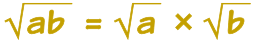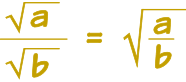# 简化平方根

### 例子：√8 可以简化为 2√2### 例子（续）

√8 = √(4×2) = √4 × √2 = 2√2

（因为 4 的平方根是 2）

### 例子：简化 √12

12 是 4 乘 3：

√12 = √(4 × 3)

√(4 × 3) = √4 × √3

4 的平方根是 2：

√4 × √3 = 2√3

### 例子：简化 √18

√18 = √(9 × 2) = √9 × √2 = 3√2

### 例子：简化 √6 × √15

√6 × √15 = √(6 × 15)

√(6 × 15) = √(2 × 3 × 3 × 5)

√(2 × 3 × 3 × 5) = √(3 × 3) × √(2 × 5) = 3√10

## 分数### 例子：简化 √30 / √10

√30 / √10 = √(30 / 10)

√(30 / 10) = √3

## 比较复杂的例子

### 例子：简化 (√20 × √5) / √2

(√20 × √5)/√2
(√(2 × 2 × 5) × √5)/√2
(√2 × √2 × √5 × √5)/√2
√2 × √5 × √5
√2 × 5
5√2

### 例子：简化 2√12 + 9√3

2√12 = 2 × 2√3 = 4√3

4√3 + 9√3 = (4+9)√3 = 13√3